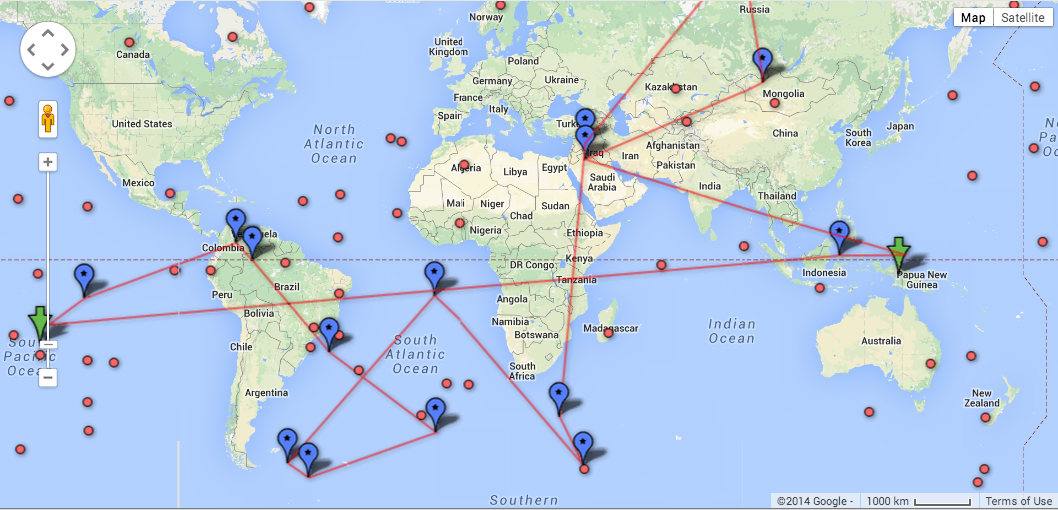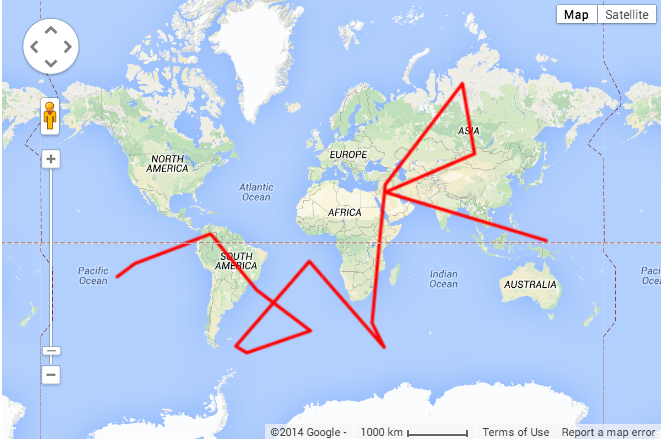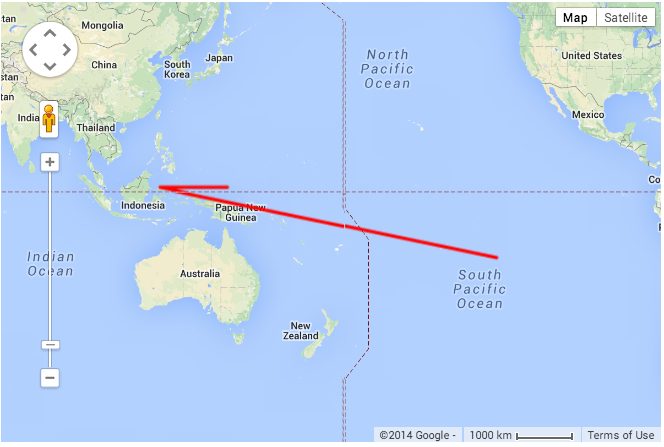# Exploring the Search Space

I moved on from sort to search and implemented a few search algorithms in python from pseudocode on wikipedia. I coded a simple backtracking algorithm as well as Dijkstra’s and an A* search.## Dijkstra’s algorithm

Dijkstra’s algorithm searches by traversing the nodes by calculating the cumulative distance to the nearest neighbor and travelling to the node with the minimum cumulative distance. The algorithm keeps track of the minimum cumulative distance calculated for each vertex.Source: Wikipedia

The brute force backtracking algorithm that I implemented overflowed the stack frame with a graph of a few thousand nodes, while Dijkstra’s algorithm had no problems. I moved onto an A* search which was familiar from my college days.A* It is essentially Dijkstra’s algorithm that chooses a path based on a heuristic evaluation of neighbors. My heuristic was to score neighbors on how close they are to a line segment that runs from the start to the finish. The math for points and lines in vector space is abstract and not so familiar. I started reading webpages on math for parametric equations and quickly moved onto this Stack Overflow thread where the code was pretty opaque and many answers incorrect. I ended up using this answer which seemed the most strait-forward.

``````1
2
3
4
5
6
7
8
9
10
11
12
13
14
15
def dist2line2(x,y,line):
x1,y1,x2,y2=line
vx = x1 - x
vy = y1 - y
ux = x2-x1
uy = y2-y1
length = ux * ux + uy * uy
det = (-vx * ux) + (-vy * uy) #//if this is < 0 or > length then its outside the line segment
if det < 0:
return (x1 - x)**2 + (y1 - y)**2
if det > length:
return (x2 - x)**2 + (y2 - y)**2
det = ux * vy - uy * vx
return det**2 / length
def dist2line(x,y,line): return math.sqrt(dist2line2(x,y,line))
``````

The resulting A* search performs really well. I chose visual testing with kml and placemarks so I could see my answer on a map. My demo code generates fake cities around the world and generates edges to randomly connect vertices.## Sources

The search output is formatted into KML which you can see on a google map (or import into google fusion tables ). The source is on Github

Updated: